## Excel VARPA Function

This post will guide you how to use the VARPA function with syntax and examples in Microsoft excel.

### Description

The Excel VARPA function returns the variance of a population based on an entire population of numbers, text, or logical values. The variance is used to identify the amount that the values vary from the average value across a set of values. And if you want to calculate the variance for a sample of the population, you can use the VARA function.

The VARPA function is a build-in function in Microsoft Excel and it is categorized as a Statistical Function.

The VARPA function is available in Excel 2016, Excel 2013, Excel 2010, Excel 2007, Excel 2003, Excel XP, Excel 2000, Excel 2011 for Mac.

### Syntax

The syntax of the VARPA function is as below:

`= VARPA (value1, [value2], ..)`

Where the VARA function arguments are:

• Value1-This is a required argument.  The first number, text or logical value or cell reference in a sample of a population.
• Value2 -This is an optional argument.  Value arguments 2 to 255 corresponding to a sample of a population. And it can only accept up to 30 number arguments in Excel 2003 or older.

Note:

• Arguments can be the following: numbers; names, arrays, or references that contain numbers; text representations of numbers; or logical values, such as TRUE and FALSE, in a reference.
• Arguments that contain TRUE evaluate as 1; arguments that contain text or FALSE evaluate as 0 (zero).
• The VARPA function is only used for its arguments are an entire population. and if the argument represents a sample of the population, you need to use the VARA function to calculate the variance.
• Arguments that are error values or text that cannot be translated into numbers cause errors.
• If the argument contain the logical values and text representations of numbers and those values will be counted.

### Excel VARPA Function Examples

The below examples will show you how to use Excel VARPA Function to calculate the variance for data that represents the entire population.

1# to get variance of an entire population of data, using the following formula:

=VARPA (A1:A2)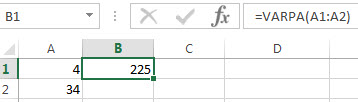### Related Functions

• Excel VARA Function
The Excel VARA function returns the variance of a population based on a sample of numbers, text, or logical values. The syntax of the VARA function is as below:= VARA (value1, [value2], ..)…
• Excel VAR Function
The Excel VAR function returns the variance of a population based on a sample of numbers. This function has been replaced by the VAR.S function in Excel 2010. The syntax of the VAR function is as below:=VAR(number1,[number2],…)…
• Excel VARP Function
The Excel VARP function returns the variance of a population based on an entire population of numbers. The syntax of the VARP function is as below:=VARP(number1,[number2],…)…

## Excel VARP Function

This post will guide you how to use the VARP function with syntax and examples in Microsoft excel.

### Description

The Excel VARP function returns the variance of a population based on an entire population of numbers. This function has been replaced by the VAR.P function in Excel 2010. The variance is used to identify the amount that the values vary from the average value across a set of values. And if you want to calculate the variance for a sample of the population, you can use the VAR function.

The VARP function is a build-in function in Microsoft Excel and it is categorized as a Statistical Function.

The VARP function is available in Excel 2016, Excel 2013, Excel 2010, Excel 2007, Excel 2003, Excel XP, Excel 2000, Excel 2011 for Mac.

### Syntax

The syntax of the VARP function is as below:

`=VARP(number1,[number2],...)`

Where the VAR function arguments are:

• number1-This is a required argument.  The first number or cell reference in a sample of a population.
• number2 -This is an optional argument.  Number arguments 2 to 255 corresponding to a sample of a population. And it can only accept up to 30 number arguments in Excel 2003 or older.

Note:

• The argument must be numbers or arrays or cell references that contain only numbers.
• The VARP function is only used for its arguments are the entire population, and if the argument represents a sample of the population, you need to use the VAR function to calculate the variance.
• If the argument contain the logical values and text representations of numbers and those values will be counted.
• Arguments that are error values or text that cannot be translated into numbers cause errors.
• VARP uses the following formula: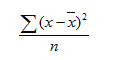### Excel VARP Function Examples

The below examples will show you how to use Excel VARP Function to calculate the variance for data that represents the entire population.

1# to get variance of an entire population of data, using the following formula:

`=VARP(A1:A2)`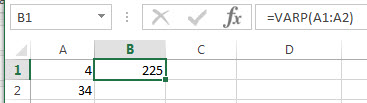### Related Functions

• Excel VARA Function
The Excel VARA function returns the variance of a population based on a sample of numbers, text, or logical values. The syntax of the VARA function is as below:= VARA (value1, [value2], ..)…
• Excel VAR Function
The Excel VAR function returns the variance of a population based on a sample of numbers. This function has been replaced by the VAR.S function in Excel 2010. The syntax of the VAR function is as below:=VAR(number1,[number2],…)…
• Excel VARPA Function
The Excel VARPA function returns the variance of a population based on an entire population of numbers, text, or logical values. The syntax of the VARPA function is as below:= VARPA (value1, [value2], ..)…

## Excel VARA Function

This post will guide you how to use the VARA function with syntax and examples in Microsoft excel.

### Description

The Excel VARA function returns the variance of a population based on a sample of numbers, text, or logical values. The variance is used to identify the amount that the values vary from the average value across a set of values. And if you want to calculate the variance for an entire population, you can use the VARP function or the newer VAR.P function.

The VARA function is a build-in function in Microsoft Excel and it is categorized as a Statistical Function.

The VARA function is available in Excel 2016, Excel 2013, Excel 2010, Excel 2007, Excel 2003, Excel XP, Excel 2000, Excel 2011 for Mac.

### Syntax

The syntax of the VARA function is as below:

`=VARA(value1, [value2], ..)`

Where the VARA function arguments are:

• Value1-This is a required argument.  The first number, text or logical value or cell reference in a sample of a population.
• Value2 -This is an optional argument.  Value arguments 2 to 255 corresponding to a sample of a population. And it can only accept up to 30 number arguments in Excel 2003 or older.

Note:

• Arguments can be the following: numbers; names, arrays, or references that contain numbers; text representations of numbers; or logical values, such as TRUE and FALSE, in a reference.
• Arguments that contain TRUE evaluate as 1; arguments that contain text or FALSE evaluate as 0 (zero).
• The VARA function is only used for its arguments are a sample of the population. And if the argument represents the entire population, you need to use the VARAP function to calculate the variance.
• Arguments that are error values or text that cannot be translated into numbers cause errors.
• If the argument contain the logical values and text representations of numbers and those values will be counted.
• VARA uses the following formula: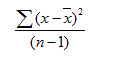### Excel VARA Function Examples

The below examples will show you how to use Excel VARA Function to calculate the variance for data that represents a sample of the population.

1# to get variance of a sample of data, using the following formula:

`=VARA(A1:A2)`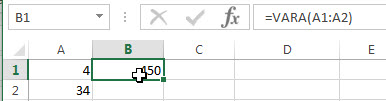### Related Functions

• Excel VAR Function
The Excel VAR function returns the variance of a population based on a sample of numbers. This function has been replaced by the VAR.S function in Excel 2010. The syntax of the VAR function is as below:=VAR(number1,[number2],…)…
• Excel VARP Function
The Excel VARP function returns the variance of a population based on an entire population of numbers. The syntax of the VARP function is as below:=VARP(number1,[number2],…)…
• Excel VARPA Function
The Excel VARPA function returns the variance of a population based on an entire population of numbers, text, or logical values. The syntax of the VARPA function is as below:= VARPA (value1, [value2], ..)…

## Excel VAR Function

This post will guide you how to use the VAR function with syntax and examples in Microsoft excel.

### Description

The Excel VAR function returns the variance of a population based on a sample of numbers. This function has been replaced by the VAR.S function in Excel 2010. The variance is used to identify the amount that the values vary from the average value across a set of values. And if you want to calculate the variance for an entire population, you can use the VARP function or the newer VAR.P function.

The VAR function is a build-in function in Microsoft Excel and it is categorized as a Statistical Function.

The VAR function is available in Excel 2016, Excel 2013, Excel 2010, Excel 2007, Excel 2003, Excel XP, Excel 2000, Excel 2011 for Mac.

### Syntax

The syntax of the VAR function is as below:

`=VAR(number1,[number2],...)`

Where the VAR function arguments are:

• number1-This is a required argument.  The first number or cell reference in a sample of a population.
• number2 -This is an optional argument.  Number arguments 2 to 255 corresponding to a sample of a population. And it can only accept up to 30 number arguments in Excel 2003 or older.

Note:

• The argument must be numbers or arrays or cell references that contain only numbers.
• The VAR function is only used for its arguments are a sample of the population. And if the argument represents the entire population, you need to use the VARP function to calculate the variance.
• If the argument contain the logical values and text representations of numbers and those values will be counted.
• Arguments that are error values or text that cannot be translated into numbers cause errors.
• VAR uses the following formula: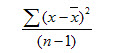### Excel VAR Function Examples

The below examples will show you how to use Excel VAR Function to calculate the variance for data that represents a sample of the population.

1# to get variance of a sample of numbers, using the following formula:

`=VAR (A1:A2)`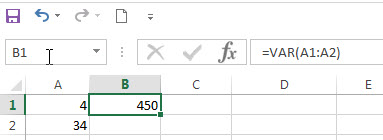### Related Functions

• Excel VARA Function
The Excel VARA function returns the variance of a population based on a sample of numbers, text, or logical values. The syntax of the VARA function is as below:= VARA (value1, [value2], ..)…
• Excel VARP Function
The Excel VARP function returns the variance of a population based on an entire population of numbers. The syntax of the VARP function is as below:=VARP(number1,[number2],…)…
• Excel VARPA Function
The Excel VARPA function returns the variance of a population based on an entire population of numbers, text, or logical values. The syntax of the VARPA function is as below:= VARPA (value1, [value2], ..)…

## Excel STDEVPA Function

This post will guide you how to use the STDEVPA function with syntax and examples in Microsoft excel.

### Description

The Excel STDEVPA function returns the standard deviation of a population based on an entire population of numbers, text or logical values. So the STDEVPA function can evaluate the text and logical values that appear in cell references or arrays. The TEXT is evaluated as zero, TRUE is evaluated as 1, and FALSE is evaluated as zero. The standard deviation is used to identify the amount that a set of values differ from the average value.

The STDEVPA function is a build-in function in Microsoft Excel and it is categorized as a Statistical Function.

The STDEVPA function is available in Excel 2016, Excel 2013, Excel 2010, Excel 2007, Excel 2003, Excel XP, Excel 2000, Excel 2011 for Mac.

### Syntax

The syntax of the STDEVPA function is as below:

`= STDEVPA (value1, [value2], ...)`

Where the STDEVPA function arguments are:

• Value1-This is a required argument.  The first number, text or logical value or cell reference in a sample of a population.
• Value2 -This is an optional argument.  Value arguments 2 to 255 corresponding to a sample of a population. And it can only accept up to 30 number arguments in Excel 2003 or older.

Note:

• Arguments can be the following: numbers; names, arrays, or references that contain numbers; text representations of numbers; or logical values, such as TRUE and FALSE, in a reference.
• Arguments that contain TRUE evaluate as 1; arguments that contain text or FALSE evaluate as 0 (zero).
• The STDEVPA function is only used for its arguments are the entire population, and if the argument represents a sample of the population, you need to use the STDEVA function to calculate the standard deviation.
• Arguments that are error values or text that cannot be translated into numbers cause errors.
• If the argument contain the logical values and text representations of numbers and those values will be counted.
• STDEVPA uses the following formula: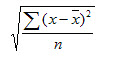### Excel STDEVPA Function Examples

The below examples will show you how to use Excel STDEVPA Function to calculate the standard deviation for data that represents the entire of population.

1# to get standard deviation of an entire population of numbers, using the following formula:

`=STDEVPA (A1:A2)`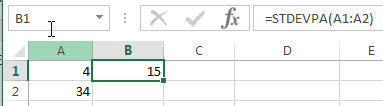### Related Functions

• Excel STDEV Function
The Excel STDEV function returns the standard deviation of a population based on a sample of numbers. This function has been replaced by the STDEV.S function in Excel 2010.The syntax of the STDEV function is as below:= STDEV (number1,[number2],…)…
• Excel STDEVA Function
The Excel STDEVA function returns the standard deviation of a population based on a sample of numbers, text, and logical values. The syntax of the STDEVA function is as below:= STDEVA (value1, [value2], ..)…
• Excel STDEVP Function
The Excel STDEVP function returns the standard deviation of a population based on an entire population of numbers. This function has been replaced by the STDEV.P function in Excel 2010.The syntax of the STDEVP function is as below:= STDEVP(number1,[number2],…)…

## Excel STDEVP Function

This post will guide you how to use the STDEVP function with syntax and examples in Microsoft excel.

### Description

The Excel STDEVP function returns the standard deviation of a population based on an entire population of numbers. This function has been replaced by the STDEV.P function in Excel 2010. The standard deviation is used to identify the amount that a set of values differ from the average value.

The STDEVP function is a build-in function in Microsoft Excel and it is categorized as a Statistical Function.

The STDEVP function is available in Excel 2016, Excel 2013, Excel 2010, Excel 2007, Excel 2003, Excel XP, Excel 2000, Excel 2011 for Mac.

### Syntax

The syntax of the STDEVP function is as below:

`= STDEVP(number1,[number2],...)`

Where the STDEVP function arguments are:

• number1-This is a required argument.  The first number or cell reference in a sample of a population.
• number2 – This is an optional argument.  Number arguments 2 to 255 corresponding to a sample of a population. and it can only accept up to 30 number arguments in Excel 2003 or older.

Note:

• The argument must be numbers or arrays or cell references that contain only numbers.
• The standard deviation is calculated using the “n” method.
• The STDEVP function is only used for its arguments are the entire population, and if the argument represents a sample of the population, you need to use the STDEVP function to calculate the standard deviation.
• If the argument contain the logical values and text representations of numbers and those values will be counted.
• Arguments that are error values or text that cannot be translated into numbers cause errors.
• STDEVP uses the following formula: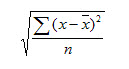### Excel STDEVP Function Examples

The below examples will show you how to use Excel STDEVP Function to calculate the standard deviation for data that represents the entire of population.

1# to get standard deviation of an entire population of numbers, using the following formula:

`=STDEVP(A1:A2)`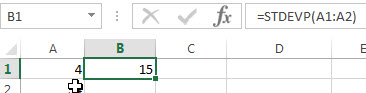### Related Functions

• Excel STDEV Function
The Excel STDEV function returns the standard deviation of a population based on a sample of numbers. This function has been replaced by the STDEV.S function in Excel 2010.The syntax of the STDEV function is as below:= STDEV (number1,[number2],…)…
• Excel STDEVA Function
The Excel STDEVA function returns the standard deviation of a population based on a sample of numbers, text, and logical values. The syntax of the STDEVA function is as below:= STDEVA (value1, [value2], ..)…
• Excel STDEVPA Function
The Excel STDEVPA function returns the standard deviation of a population based on an entire population of numbers, text or logical values. The syntax of the STDEVPA function is as below:= STDEVPA (value1, [value2], …)…

## Excel STDEVA Function

This post will guide you how to use the STDEVA function with syntax and examples in Microsoft excel.

### Description

The Excel STDEVA function returns the standard deviation of a population based on a sample of numbers, text, and logical values. So the STDEVA function can evaluate the text and logical values that appear in cell references or arrays. The TEXT is evaluated as zero, TRUE is evaluated as 1, and FALSE is evaluated as zero. The standard deviation is used to identify the amount that a set of values differ from the average value. And if you want to calculate the standard deviation for an entire population, you can use the STDEVP or STDEV.P function.

The STDEVA function is a build-in function in Microsoft Excel and it is categorized as a Statistical Function.

The STDEVA function is available in Excel 2016, Excel 2013, Excel 2010, Excel 2007, Excel 2003, Excel XP, Excel 2000, Excel 2011 for Mac.

### Syntax

The syntax of the STDEVA function is as below:

`= STDEVA (value1, [value2], ..)`

Where the STDEVA function arguments are:

• Value1-This is a required argument.  The first number, text or logical value or cell reference in a sample of a population.
• Value2 -This is an optional argument.  Value arguments 2 to 255 corresponding to a sample of a population. And it can only accept up to 30 number arguments in Excel 2003 or older.

Note:

• Arguments can be the following: numbers; names, arrays, or references that contain numbers; text representations of numbers; or logical values, such as TRUE and FALSE, in a reference.
• Arguments that contain TRUE evaluate as 1; arguments that contain text or FALSE evaluate as 0 (zero).
• The STDEVA function is only used for its arguments are a sample of the population. and if the argument represents the entire population, you need to use the STDEVP function to calculate the standard deviation.
• Arguments that are error values or text that cannot be translated into numbers cause errors.
• If the argument contain the logical values and text representations of numbers and those values will be counted.
• STDEVA uses the following formula: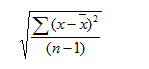### Excel STDEVA Function Examples

The below examples will show you how to use Excel STDEVA Function to calculate the standard deviation for data that represents a sample of the population.

1# to get standard deviation of a sample of numbers, using the following formula:

=STDEVA (A1:A2)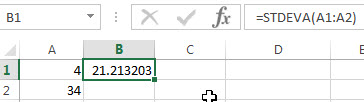### Related Functions

• Excel STDEV Function
The Excel STDEV function returns the standard deviation of a population based on a sample of numbers. This function has been replaced by the STDEV.S function in Excel 2010.The syntax of the STDEV function is as below:= STDEV (number1,[number2],…)…
• Excel STDEVP Function
The Excel STDEVP function returns the standard deviation of a population based on an entire population of numbers. This function has been replaced by the STDEV.P function in Excel 2010.The syntax of the STDEVP function is as below:= STDEVP(number1,[number2],…)…
• Excel STDEVPA Function
The Excel STDEVPA function returns the standard deviation of a population based on an entire population of numbers, text or logical values. The syntax of the STDEVPA function is as below:= STDEVPA (value1, [value2], …)…

## Excel STDEV Function

This post will guide you how to use the STDEV function with syntax and examples in Microsoft excel.

### Description

The Excel STDEV function returns the standard deviation of a population based on a sample of numbers. This function has been replaced by the STDEV.S function in Excel 2010. The standard deviation is used to identify the amount that a set of values differ from the average value. And if you want to calculate the standard deviation for an entire population, you can use the STDEVP or STDEV.P function.

The STDEV function is a build-in function in Microsoft Excel and it is categorized as a Statistical Function.

The STDEV function is available in Excel 2016, Excel 2013, Excel 2010, Excel 2007, Excel 2003, Excel XP, Excel 2000, Excel 2011 for Mac.

### Syntax

The syntax of the STDEV function is as below:

`= STDEV (number1,[number2],...)`

Where the STDEV function arguments are:

• number1-This is a required argument.  The first number or cell reference in a sample of a population.
• number2 -This is an optional argument.  Number arguments 2 to 255 corresponding to a sample of a population. And it can only accept up to 30 number arguments in Excel 2003 or older.

Note:

• The argument must be numbers or arrays or cell references that contain only numbers.
• The STDEV function is only used for its arguments are a sample of the population. And if the argument represents the entire population, you need to use the STDEVP function to calculate the standard deviation.
• If the argument contain the logical values and text representations of numbers and those values will be counted.
• STDEV uses the following formula:

### Excel STDEV Function Examples

The below examples will show you how to use Excel STDEV Function to calculate the standard deviation for data that represents a sample of the population.

1# to get standard deviation of a sample of numbers, using the following formula:

`=STDEV(A1:A2)`

### Related Functions

• Excel STDEVA Function
The Excel STDEVA function returns the standard deviation of a population based on a sample of numbers, text, and logical values. The syntax of the STDEVA function is as below:= STDEVA (value1, [value2], ..)…
• Excel STDEVP Function
The Excel STDEVP function returns the standard deviation of a population based on an entire population of numbers. This function has been replaced by the STDEV.P function in Excel 2010.The syntax of the STDEVP function is as below:= STDEVP(number1,[number2],…)…
• Excel STDEVPA Function
The Excel STDEVPA function returns the standard deviation of a population based on an entire population of numbers, text or logical values. The syntax of the STDEVPA function is as below:= STDEVPA (value1, [value2], …)…

## Excel SLOPE Function

This post will guide you how to use the SLOPE function with syntax and examples in Microsoft excel.

### Description

The Excel SLOPE function returns the slope of the linear regression line through data points in known_y’s and know_x’s. So you can use this function to calculate the slope of the linear regression line through a given set of x-values and y-values in Excel.

The SLOPE function is a build-in function in Microsoft Excel and it is categorized as a Statistical Function.

The SLOPE function is available in Excel 2016, Excel 2013, Excel 2010, Excel 2007, Excel 2003, Excel XP, Excel 2000, Excel 2011 for Mac.

### Syntax

The syntax of the SLOPE function is as below:

`= SLOPE (known_y's, known_x's)`

Where the SLOPE function arguments are:

• known_y’s -This is a required argument.  An array or cell reference that contain numeric known y-values used to calculate the slope.
• known_x’s -This is a required argument.  An array or cell reference that contain numeric known x-values used to calculate the slope.

Note:

• The argument must be numbers or arrays or cell references that contain only numbers.
• If any arguments contain text, logical values, or empty cells, those values will be ignored by the SLOPE function.
• If known_y’s and known_x’s arguments have a different lengths, the SLOPE function will return the #N/A Error.
• The equation for the slope of the regression line is: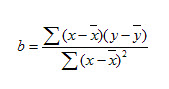### Excel SLOPE Function Examples

The below examples will show you how to use Excel SLOPE Function to calculate the slope of the linear regression line through a given set of values.

1# to calculate the slope of the linear regression line through the data points in A1:A4 and B1:B4, using the following formula:

`=SLOPE (A1:A4,B1:B4)`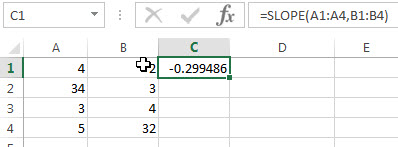## Excel RANK Function

This post will guide you how to use the RANK function with syntax and examples in Microsoft excel.

### Description

The Excel RANK function returns the rank of a given number in a supplied range of cells.  If there are duplicated values in a list of values, it will be set the same rank. This function has been replaced by the RANK.EQ function in Excel 2010.

The RANK function is a build-in function in Microsoft Excel and it is categorized as a Statistical Function.

The RANK function is available in Excel 2016, Excel 2013, Excel 2010, Excel 2007, Excel 2003, Excel XP, Excel 2000, Excel 2011 for Mac.

### Syntax

The syntax of the RANK function is as below:

`= RANK (number,ref,[order])`

Where the RANK function arguments are:

• Number – This is a required argument.  The number for which you want to get the rank.
• ref – This is a required argument. An array or range of cells, or cell reference that contain the supplied number.
• Order – This is an optional argument. . A number specifying how to rank number. If order is 0 or omitted, the RANK function will rank the numbers in descending order. If the order is not equal to 0, the RANK function will rank numbers in ascending order.

### Excel RANK Function Examples

The below examples will show you how to use Excel RANK Function to get the rank of a given value within a supplied range of cells.

Example 1:  to get the rank of 4 in a given range of cells (A1:A4), using the following formula:

`=RANK(5,A1:A4,1)`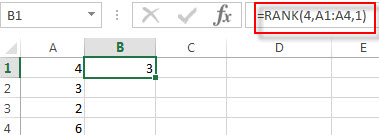### More Excel Rank Function Examples

• Rank Data with Multiple Criteria
Assuming that you want to rank data against multiple criteria for a range of cells in your worksheet. How to achieve it. You need to create a new complex formula based on the SUMPRODUCT function. .…
•  Rank values in a column based a specific value in another column
Assuming that you have a list of data contains two columns and the first column is product list and another is Sales number. You want to rank the sales number of a specified product name. You can try to write a complex formula based on the IF function and the COUNTIFS function to achieve the result..…
• Reverse Rank Order
Assuming that you have a formula based on RANK function that ranks numbers by biggest to smallest by default. But you want to go the opposite from the smallest to biggest.…
• Rank data Based on the Number of Occurrences
You need to get the number of occurrences for the selected range of cells, then rank those number using the RANK function…

## Excel QUARTILE Function

This post will guide you how to use the QUARTILE function with syntax and examples in Microsoft excel.

### Description

The Excel QUARTILE function returns the quartile from a supplied range of values. This function has been replaced with QUARTILE.EXC function and QUARTILE.INC function.

The QUARTILE function is a build-in function in Microsoft Excel and it is categorized as a Statistical Function.

The QUARTILE function is available in Excel 2016, Excel 2013, Excel 2010, Excel 2007, Excel 2003, Excel XP, Excel 2000, Excel 2011 for Mac.

### Syntax

The syntax of the QUARTILE function is as below:

`= QUARTILE (array,quart)`

Where the QUARTILE function arguments are:

• Array – This is a required argument.  The array or range of cells that contain numeric values for which you want to get the quartile value.
• Quart – This is a required argument. The quartile value that you want to return.  The value can be set in the following 5 values.
 If quart equals QUARTILE returns 0 Minimum value 1 First quartile (25th percentile) 2 Median value (50th percentile) 3 Third quartile (75th percentile) 4 Maximum value

Note:

• If the quart value is not an integer, and the QUARTILE function will truncate it.
• If the quart argument is empty, the QUARTILE function will return the #NUM! Error.
• If the quart value is small than 0 or greater than 4, the QUARTILE function will return the #NUM! Error.

### Excel QUARTILE Function Examples

The below examples will show you how to use Excel QUARTILE Function to get the quartile from a range of cells.

Example 1:  to get the first quartile of a given range of cells (A1:A4), using the following formula:

`= QUARTILE(A1:A4,1)`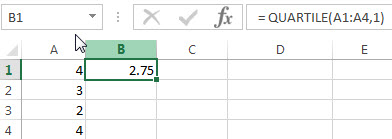## Excel PERMUT Function

This post will guide you how to use the PERMUT function with syntax and examples in Microsoft excel.

### Description

The Excel PERMUT function returns the number of permutations for a given number of items.

The PERMUT function is a build-in function in Microsoft Excel and it is categorized as a Statistical Function.

The PERMUT function is available in Excel 2016, Excel 2013, Excel 2010, Excel 2007, Excel 2003, Excel XP, Excel 2000, Excel 2011 for Mac.

### Syntax

The syntax of the PERMUT function is as below:

`=PERMUT(number,number_chosen])`

Where the PERMUT function arguments are:

• Number – This is a required argument.  An integer that describes the number of objects. And the total number of items.
• Number_chosen – This is a required argument. The number of objects in each permutation.

Note:

• If both number and number_chosen arguments are non-numeric, the PERMUT function will return the #VALUE! Error.
• If the number argument is small than 0 or equal to 0, the PERMUT function will return the #NUM! Error.
• If the number value is small than number_chosen, the PERMUT function will return the #NUM! Error.
• The equation for the number of permutations is: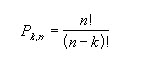Excel PERMUT Function Examples

The below examples will show you how to use Excel PERMUT Function to get the number of permutations of a given number of items.

Example 1:  to get permutations possible for a group of 6 objects where 3 are chosen, using the following formula:

= PERMUT (6,3)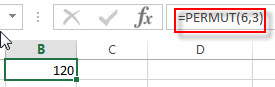## Excel CHITEST Function

This post will guide you how to use the CHITEST function with syntax and examples in Microsoft excel.

### Description

The Excel CHITEST function returns the value from the chi-squared distribution for the statistic and the appropriate degrees of freedom. So you can the chi-square test to calculate the probability that the differences between two given date sets in Excel.

The CHITEST function is a build-in function in Microsoft Excel and it is categorized as a Statistical Function.

The CHITEST function is available in Excel 2016, Excel 2013, Excel 2010, Excel 2007, Excel 2003, Excel XP, Excel 2000, Excel 2011 for Mac.

### Syntax

The syntax of the CHITEST function is as below:

`= CHITEST(actual_range, expected_range)`

Where the CHITEST function arguments are:

• Actual_range -This is a required argument. The actual range of data to test against expected range.
• expected_range – This is a required argument.  The range of data that contains the ratio of the product of row totals and column totals to the grand total.

Note:

• If actual_range and expected_range have a different number of data points, CHITEST returns the #N/A error value.

### Excel CHITEST Function Examples

The below examples will show you how to use Excel CHITEST Function to get the value from the chi-squared distribution.

1# to calculate the right-tailed probability of the chi-square distribution, using the following formula:

`= CHITEST(A1:A2,B1:B2)`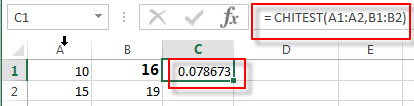### Related Functions

• Excel CHIDIST function
The Excel CHIDIST function returns the right-tailed probability of the chi-squared distribution.The syntax of the CHIDIST function is as below:= CHIDIST (x,deg_freedom).…
• Excel CHIINV function
The Excel CHIINV function returns the inverse of the right-tailed probability of the chi-squared distribution.  For example, if the probability is equal to CHIDIST(x,…), then the CHIINV(probability,…) is equal to x.The syntax of the CHIINV function is as below:= CHIINV (probability, deg_freedom).…

## Excel CHIINV function

This post will guide you how to use the CHIINV function with syntax and examples in Microsoft excel.

### Description

The Excel CHIINV function returns the inverse of the right-tailed probability of the chi-squared distribution.  For example, if the probability is equal to CHIDIST(x,…), then the CHIINV(probability,…) is equal to x.

The CHIINV function is a build-in function in Microsoft Excel and it is categorized as a Statistical Function.

The CHIINV function is available in Excel 2016, Excel 2013, Excel 2010, Excel 2007, Excel 2003, Excel XP, Excel 2000, Excel 2011 for Mac.

### Syntax

The syntax of the CHIINV function is as below:

`= CHIINV (probability, deg_freedom)`

Where the CHIINV function arguments are:

• Probability -This is a required argument. The probability for the chi-squared distribution.
• deg_freedom – This is a required argument.  The number of degrees of freedom. (the value must be a positive integer)

Note:

• If any argument is non-numeric, the CHIINV function will return #VALUE! Error.
• If deg_freedom argument is not integer and the CHIINV function will truncate it.
• If deg_freedom argument is smaller than 1, the CHIINV function will return the #NUM! Error.
• If probability is smaller than 0 or greater than 1, then the CHIINV function will return the #NUM! Error.

### Excel CHIINV Function Examples

The below examples will show you how to use Excel CHIINV Function to get the inverse of the right-tailed probability of the chi-squared distribution.

1# to calculate the right-tailed probability of the chi-square distribution, using the following formula:

=CHIINV(0.06,10)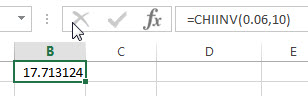### Related Functions

• Excel CHIDIST function
The Excel CHIDIST function returns the right-tailed probability of the chi-squared distribution.The syntax of the CHIDIST function is as below:= CHIDIST (x,deg_freedom).…
• Excel CHITEST Function
The Excel CHITEST function returns the value from the chi-squared distribution for the statistic and the appropriate degrees of freedom. The syntax of the CHITEST function is as below:= CHITEST(actual_range, expected_range)…

## Excel CHIDIST function

This post will guide you how to use the CHIDIST function with syntax and examples in Microsoft excel.

### Description

The Excel CHIDIST function returns the right-tailed probability of the chi-squared distribution.

The CHIDIST function is a build-in function in Microsoft Excel and it is categorized as a Statistical Function.

The CHIDIST function is available in Excel 2016, Excel 2013, Excel 2010, Excel 2007, Excel 2003, Excel XP, Excel 2000, Excel 2011 for Mac.

### Syntax

The syntax of the CHIDIST function is as below:

`= CHIDIST (x,deg_freedom)`

Where the CHIDIST function arguments are:

• X -This is a required argument. The value at which you want to determine the chi-squared distribution.
• deg_freedom – This is a required argument.  The number of degrees of freedom. (the value must be an integer between 1 and 10^10)

Note:

• If any argument is non-numeric, the CHIDIST function will return #VALUE! Error.
• If deg_freedom argument is not integer and the CHIDIST function will truncate it.
• If x is negative, the CHIDIST function will return the #NUM! Error.
• If deg_freedom argument is smaller than 1 or greater than 10^10, the CHIDIST function will return the #NUM! Error.

### Excel CHIDIST Function Examples

The below examples will show you how to use Excel CHIDIST Function to get one-tailed probability of the chi-squared distribution.

1# to calculate the right-tailed probability of the chi-square distribution, using the following formula:

`=CHIDIST(16.4,10)`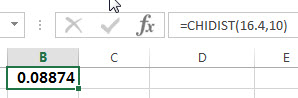### Related Functions

• Excel CHIINV function
The Excel CHIINV function returns the inverse of the right-tailed probability of the chi-squared distribution.  For example, if the probability is equal to CHIDIST(x,…), then the CHIINV(probability,…) is equal to x.The syntax of the CHIINV function is as below:= CHIINV (probability, deg_freedom).…

## Excel BINOM.INV function

This post will guide you how to use the BINOM.INV function with syntax and examples in Microsoft excel.

### Description

The Excel BINOM.INV function returns the inverse of the Cumulative Binomial Distribution that is greater than or equal to a criterion value. And this function will return the smallest value for which the cumulative binomial distribution.

The BINOM.INV function is a build-in function in Microsoft Excel and it is categorized as a Statistical Function.

The BINOM.INV function is available in Excel 2016, Excel 2013, Excel 2010, Excel 2011 for Mac.

### Syntax

The syntax of the BINOM.INV function is as below:

`=BINOM.INV(trials, probability_s, alpha)`

Where the BINOM.INV function arguments are:

• Trials – This is a required argument.  The number of Bernoulli trials.
• probability_s – This is a required argument.  The probability of success for each trial.
• Alpha – This is a required argument. The criterion value. The value must be 0 or 1.

Note:

• If any argument is non-numeric, the BINOM.INV function will return #VALUE! Error.
• If trials argument is not integers and the BINOM.INV function will truncate it.
• If trials argument is smaller than 0, the BINOM.INV function will return the #NUM! Error.
• If probability_s is small than 0 or greater than 1, then the BINOM.INV function will return the #NUM! Error.
• If alpha argument is smaller than 0 or greater than 1, then the BINOM.INV function will return the #NUM! Error.

### Excel BINOM.INV Function Examples

The below examples will show you how to use Excel BINOM.INV Function to get the inverse of the Cumulative Binomial Distribution.

Example 1: to get the smallest value for which the cumulative binomial distribution is greater than or equal to a criterion value, using the following formula:

`= BINOM.INV (5,0.6,0.55)`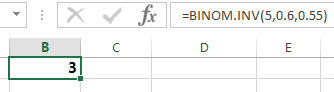### Related Functions

• Excel BINOMDIST function
The Excel BINOMDIST function returns the individual term binomial distribution probability. The syntax of the BINOMDIST function is as below:= BINOMDIST (number_s, trials, probability_s, cumulative).…
• Excel BINOM.DIST function
The Excel BINOM.DIST function returns the individual term binomial distribution probability. The syntax of the BINOM.DIST function is as below:= BINOM.DIST(number_s, trials, probability_s, cumulative).…

## Excel BINOMDIST Function

This post will guide you how to use the BINOMDIST function with syntax and examples in Microsoft excel.

### Description

The Excel BINOMDIST function returns the individual term binomial distribution probability. For example, you can use the BINOMDIST function to calculate the probability that two of the next three babies born are male in Excel. And this function has been replaced with another new function BINOM.DIST.

The BINOMDIST function is a build-in function in Microsoft Excel and it is categorized as a Statistical Function.

The BINOMDIST function is available in Excel 2016, Excel 2013, Excel 2010, Excel 2007, Excel 2003, Excel XP, Excel 2000, Excel 2011 for Mac.

### Syntax

The syntax of the BINOMDIST function is as below:

`= BINOMDIST (number_s, trials, probability_s, cumulative)`

Where the BINOMDIST function arguments are:

• number_s -This is a required argument. The number of successes that you want to calculate the probability.
• Trials – This is a required argument.  The number of independent trials.
• probability_s – This is a required argument.  The probability of success for each trial.
• Cumulative – This is a required argument. It is a logical value that specifies the type of binomial distribution to be used. If the cumulative is TRUE, the BINOMDIST function returns the cumulative distribution function; if it is equal to FALSE, the BINOMDIST function returns the probability mass function.

Note:

• If number_s, trials, or probability_s argument is non-numeric, the BINOMDIST function will return #VALUE! Error.
• If number_s and trials arguments are not integers and the BINOMDIST function will truncate it.
• If number_s argument is smaller than 0 or greater than trials argument, the BINOMDIST function will return the #NUM! Error.
• If probability_s is small than 0 or greater than 1, then the BINOMDIST function will return the #NUM! Error.

### Excel BINOMDIST Function Examples

The below examples will show you how to use Excel BINOMDIST Function to get the binomial distribution probability for a given number of successes.

Example 1: to calculate the individual term binomial distribution probability for a given number of successes from a specified number of trials, using the following formula:

=BINOMDIST(6,11,0.5,TRUE)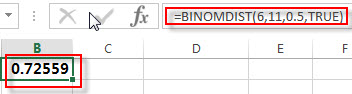### Related Functions

• Excel BINOM.DIST function
The Excel BINOM.DIST function returns the individual term binomial distribution probability. The syntax of the BINOM.DIST function is as below:= BINOM.DIST(number_s, trials, probability_s, cumulative).…
• Excel BETA.DIST function
The Excel BETA.DIST function can be used to calculate the cumulative beta distribution or beta probability density function.The syntax of the BETA.DIST function is as below:= BETA.DIST(x, alpha, beta, cumulative, [A], [B]).…
The Excel BETADIST function returns the cumulative beta probability density function.The syntax of the BETADIST function is as below:= BETADIST(x, alpha, beta, [A], [B]).…

## Excel PERCENTRANK Function

This post will guide you how to use the PERCENTRANK function with syntax and examples in Microsoft excel.

### Description

The Excel PERCENTRANK function returns the rank of a value in a set of values as a percentage of the set. So you can use the PERCENTRANK function to calculate the relative position of a value in a set of values as a percentage in Excel. This function has been replaced by the PERCENTRANK .INC function in Excel 2010.

The PERCENTRANK function is a build-in function in Microsoft Excel and it is categorized as a Statistical Function.

The PERCENTRANK function is available in Excel 2016, Excel 2013, Excel 2010, Excel 2007, Excel 2003, Excel XP, Excel 2000, Excel 2011 for Mac.

### Syntax

The syntax of the PERCENTRANK function is as below:

`= PERCENTRANK (array,x,[significance])`

Where the PERCENTRANK function arguments are:

• Array – This is a required argument.  A range or array or cell reference from which you want to get the rank of a specific value.
• X – This is a required argument. The value that you want to find the rank for.
• Significance -This is an optional argument.  A value that identifies the number of significant digits for the returned percentage value. If omitted, PERCENTRANK uses three digits (0.xxx).

Note:

• If the array argument is empty, the PERCENTRANK function will return the #VALUE! Error.
• If the significance argument is small than 1, the PERCENTRANK function will return the #NUM! Error.
• If x does not match one of the values in the array, the PERCENTRANK function will interpolate to find the percentage rank.

### Excel PERCENTRANK Function Examples

The below examples will show you how to use Excel PERCENTRANK Function to get the rank of a value in a data set as a percentage of the data set.

Example 1:  to get the percent rank of 3 in the range A1:A5, using the following formula:

`= PERCENTRANK (A1:A5)`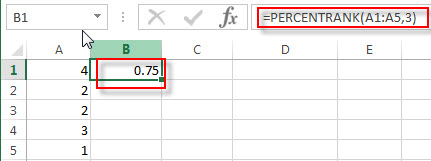Because there are 3 values are less than 3, and 1 value is greater than 3, so the percent rank of 3 in the range A1:A5 is: 3/(3+1)=0.75

Example 2:  to get the percent rank of 4 in the range A1:A5, using the following formula:

`= PERCENTRANK (A1:A5)`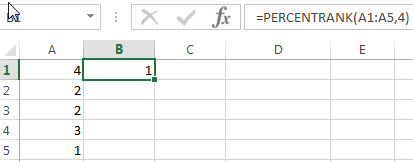Because there are 4 values are less than 4, and 0 value is greater than 4, so the percent rank of 4 in the range A1:A5 is: 4/(4+0)=0.75

## Excel PERCENTILE Function

This post will guide you how to use the PERCENTILE function with syntax and examples in Microsoft excel.

### Description

The Excel PERCENTILE function returns the kth percentile from a supplied range of values. So you can use the PERCENTILE function to determine the 30% percentile in a range in Excel. This function has been replaced by the PERCENTILE.INC function in Excel 2010.

The PERCENTILE function is a build-in function in Microsoft Excel and it is categorized as a Statistical Function.

The PERCENTILE function is available in Excel 2016, Excel 2013, Excel 2010, Excel 2007, Excel 2003, Excel XP, Excel 2000, Excel 2011 for Mac.

### Syntax

The syntax of the PERCENTILE function is as below:

`= PERCENTILE (array,k)`

Where the PERCENTILE function arguments are:

• array – This is a required argument.  A range or array or cell reference that you want to get the kth percentile.
• K – This is a required argument. The percentile value. And it can be a value between 0 and 1.

Note:

• If K argument is non-numeric, the PERCENTILE function will return the #VALUE! Error.
• If K is small than 0 or greater than 1, the PERCENTILE function will return the #NUM! Error.
• If k is not a multiple of 1/(n – 1), PERCENTILE interpolates to determine the value at the k-th percentile.

### Excel PERCENTILE Function Examples

The below examples will show you how to use Excel PERCENTILE Function to get the k-th percentile of values for a set of data.

Example 1:  to get the 50th percentile of the values in the range A1:A4, using the following formula:

`= PERCENTILE(A1:A4,0.5)`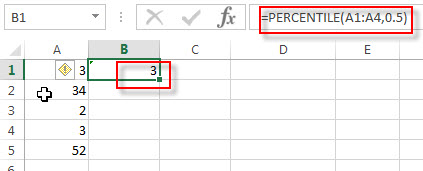## Excel MODE.SNGL Function

This post will guide you how to use the MODE.SNGL function with syntax and examples in Microsoft excel.

### Description

The Excel MODE.SNGL function returns the most frequently occurring number found in an array or range of numbers. If there are two or more frequently occurring numbers in a range of data, it will return the lowest of these numbers. The MODE.SNGL function is a new function in Excel 2010 and it is not available in earlier versions of excel, so if you are using Excel 2007 or older version, please use the MODE function instead.

The MODE.SNGL function is a build-in function in Microsoft Excel and it is categorized as a Statistical Function.

The MODE.SNGL function is available in Excel 2016, Excel 2013, Excel 2010, Excel 2011 for Mac.

### Syntax

The syntax of the MODE.SNGL function is as below:

`= MODE.SNGL(number1, [number2], ...)`

Where the MODE.SNGL function arguments are:

• number1 – This is a required argument.  A number or array or cell reference that contain only numeric values that you want to calculate the mode.
• number2 – This is an optional argument.  A number or array or reference that refers to numeric values. It can be up to 255 numeric values in Excel 2007 or later. And it only can be up to 30 number arguments in Excel 2003.

Note:

• Any arguments can either be numeric values or Defined names, arrays, or cell reference that contain only numeric values.
• If the list of number arguments contain logical values, text, or empty cells, those values will be ignored.
• If the supplied numbers in a range of data do not contain any duplicate numbers, the MODE.SNGL function will return the #N/A error.

### Excel MODE.SNGL Function Examples

The below examples will show you how to use Excel MODE.SNGL Function to the most frequently occurring value in a range of data.

Example 1:  to get the most frequently occurring number found in a range of cells A1:A5, using the following formula:

`= MODE.SNGL(A1:A5)`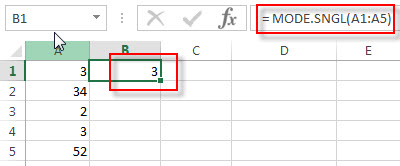### Related Functions

• Excel MEDIAN Function
The Excel MEDIAN function returns the median of the given numbers. And the median is the number in the middle of a list of supplied numbers.  The syntax of the MEDIAN function is as below:= MEDIAN (number1, [number2], …)…
• Excel MODE Function
The Excel MODE function returns the most frequently occurring number found in an array or range of numbers. If there are two or more frequently occurring numbers in a range of data, it will return the lowest of these numbers.The syntax of the MODE function is as below:= MODE(number1, [number2], …)…
• Excel MODE.MULT Function
The Excel MODE.MULT function returns a vertical array of the most frequently occurring number found in an array or range of numbers. The syntax of the MODE.MULT function is as below:= MODE.MULT (number1, [number2], …)…